# [译] Transformer 是如何工作的：600 行 Python 代码实现两个（文本分类+文本生成）Transformer（2019）

Published at 2023-06-06 | Last Update 2023-08-12

# 摘要

Transformer 是一类非常令人着迷的机器学习架构（a family of machine learning architectures）。 之前已经有一些不错的介绍文章（例如 [1, 2]），但过去几年 transformer 变得简单了很多， 因此要解释清楚现代架构（modern architectures）是如何工作的，比以前容易多了。

• 这个讲座 介绍了神经网络的基础知识；
• 这个讲座 介绍了神经网络如何应用于现代深度学习系统。

# 1 self-attention（自注意力）模型

self-attention 运算是所有 transformer 架构的基本运算

## 1.1 输入输出：vector-to-vector 运算

Self-attention 是一个 sequence-to-sequence 运算： 输入一个向量序列（a sequence of vectors），输出另一个向量序列。

$\y_\rc{i} = \sum_{\gc{j}} w_{\rc{i}\gc{j}} \x_\gc{j}$

## 1.2 权重矩阵计算和归一化

$w'_{\rc{i}\gc{j}} = {\x_\rc{i}}^T\x_\gc{j}$

$w_{\rc{i}\gc{j}} = \frac{\text{exp } w'_{\rc{i}\gc{j}}}{\sum_\gc{j} \text{exp }w'_{\rc{i}\gc{j}}}$

## 1.3 直观展示与小结

1. vector-to-vector 运算：self-attention 是对 input vector 做矩阵运算，得到一个加权结果作为 output vector；
2. 加权矩阵计算：权重矩阵不是常量，而是跟它所在的位置 (i,j) 直接相关，根据对应位置的 input vector 计算。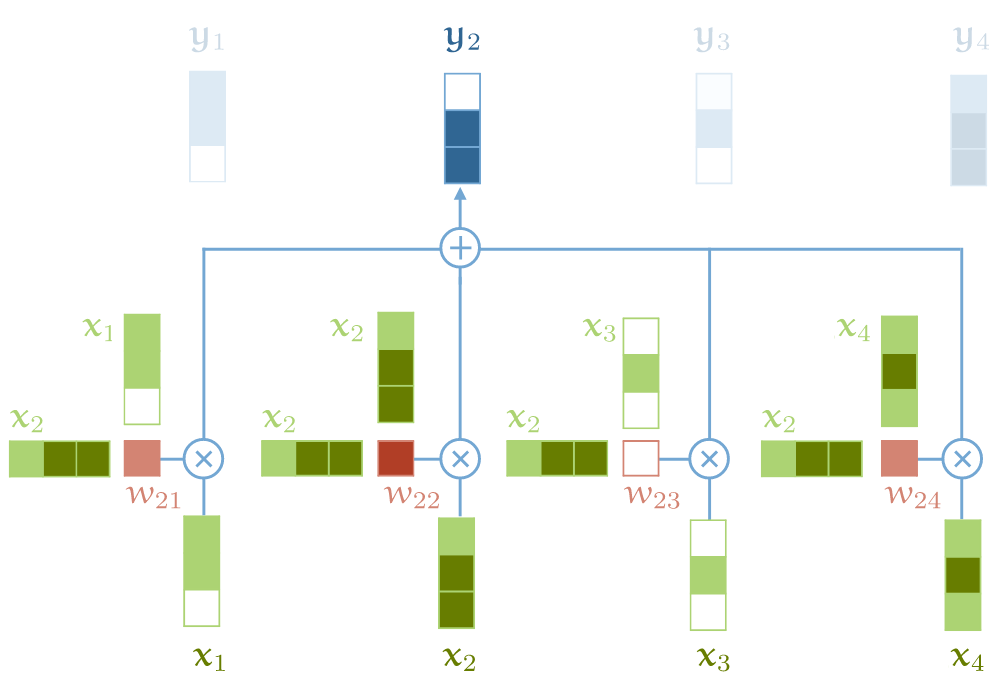self-attention 基本运算

• output vector 中的每个元素 $y_j$ 都是对 input vector 中所有元素的加权和；
• 对于 $y_j$，加权矩阵由 input 元素 $x_j$ 与每个 input 元素计算得到；

• 这是整个架构中，唯一在 input & output vector 之间 所做的运算；
• Transformer 架构中的其他运算都是单纯对 input vector 做运算

# 2 self-attention 为什么有效？以电影推荐为例

## 2.1 传统推荐系统：特性向量点积用户偏好

1. 人工设计一些电影特征，比如浪漫指数、动作指数，
2. 人工设计一些用户特征，例如他们喜欢浪漫电影或动作片的可能性；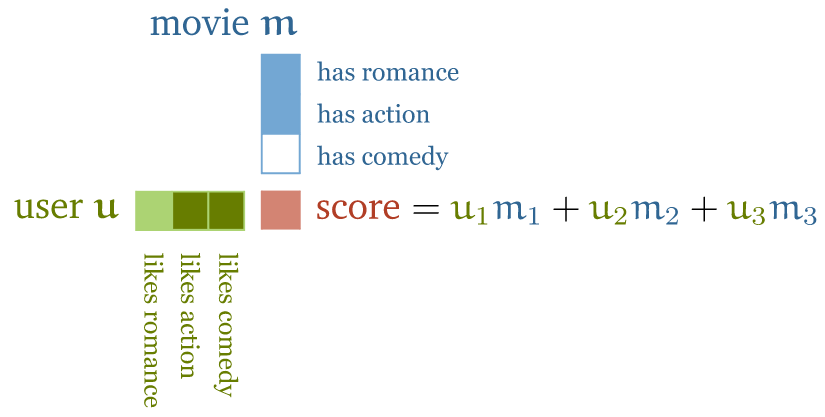• 如果特征的符号相同，例如“浪漫电影 && 用户喜欢浪漫电影”， 或者“不是浪漫电影 && 用户不喜欢浪漫电影”，得到的点积就是正数；反之就是负数；
• 特征值的大小决定该特征对总分的贡献大小： 一部电影可能有点浪漫，但不是很明显，或者用户可能只是不喜欢浪漫，但也没到讨厌的程度。

## 2.2 基于 self-attention 的推荐系统

### 2.2.1 电影特征和用户特征作为模型参数，匹配已知的用户偏好

1. 电影特征和用户特征不再直接做点积运算，而是作为模型的参数（parameters of the model）；
2. 收集少量的用户偏好作为目标，然后通过优化用户特征和电影特征（模型参数）， 使二者的点积匹配已知的用户喜好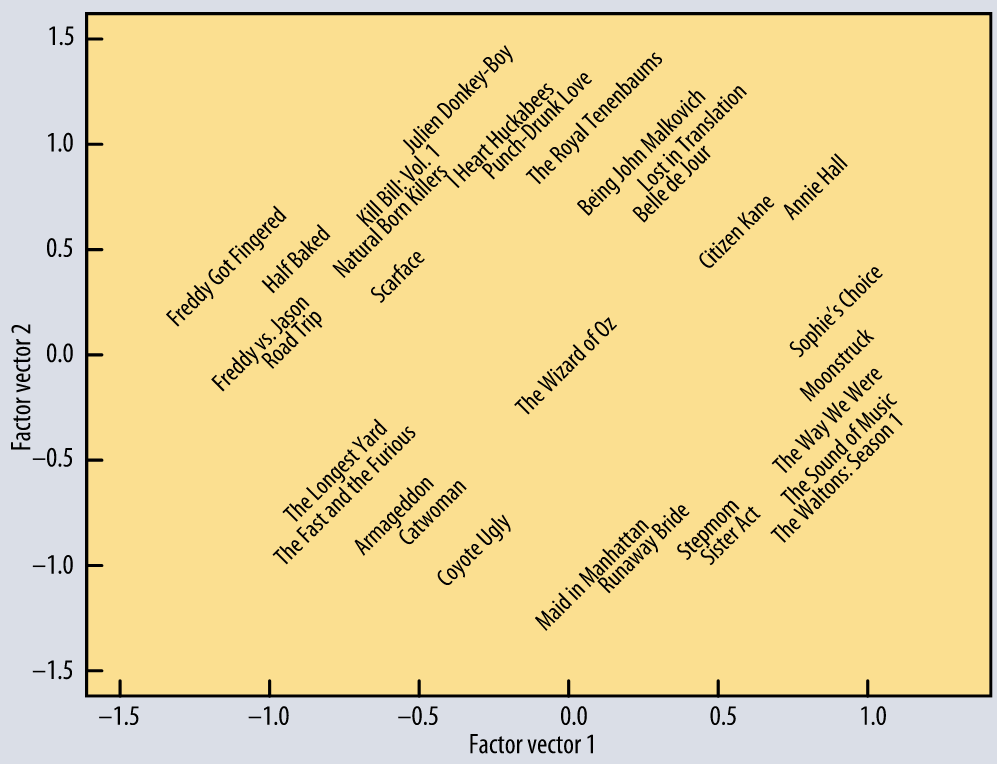### 2.2.2 嵌入层：对输入进行处理

$\bc{\text{the}}, \bc{\text{cat}}, \bc{\text{walks}}, \bc{\text{on}}, \bc{\text{the}}, \bc{\text{street}}$

$\v_\bc{\text{the}}, \v_\bc{\text{cat}}, \v_\bc{\text{walks}}, \v_\bc{\text{on}}, \v_\bc{\text{the}}, \v_\bc{\text{street}}$

$\y_\bc{\text{the}}, \y_\bc{\text{cat}}, \y_\bc{\text{walks}}, \y_\bc{\text{on}}, \y_\bc{\text{the}}, \y_\bc{\text{street}}$

### 2.2.3 直观解释

• 在大多数情况下，定冠词 "the" 与句子中其他单词表示什么意思（the interpretation of the other words）关系不大； 因此我们最终得到的嵌入层 $\v_\bc{\text{the}}$ 与所有其他单词的点积可能很小或为负数
• 另一方面，要解释这句话中 “walks” 的意思，弄清楚谁在走路是非常有用的。这很可能由名词表达， 因此对于像 cat 这样的名词和像 walks 这样的动词，我们可能最终学习到的 $\v_\bc{\text{cat}}$ and $\v_\bc{\text{walks}}$ 点积是个较大的正数

2. 输出向量是整个输入序列的加权和，权重由这些点积决定

### 2.2.4 self-attention 特殊属性

1. 到目前为止，我们的 self-attention 模型还没有参数（ 虽然下文中，我们还是会为 self-attention 添加几个参数）。

换句话说，基本的 self-attention 实际上做什么完全取决于生成输入序列的上游机制。 例如嵌入层这种机制会驱动着 self-attention 学习基于点积的表示。

2. self-attention 将输入当做一个集合（set）而不是序列（sequence）

如果我们对输入序列进行重排（permute），输出序列除了也跟着重排，其他方面将完全相同， 也就是说 self-attention 是排列等变的（permutation equivariant）。 后面会看到，构建完整的 transformer 时，我们还是会引入一些东西来保持输入的顺序信息， 但要明白 self-attention 本身是不关心输入的顺序属性的（sequential nature）。

# 3. 实现一个基本的 self-attention

What I cannot create, I do not understand. —— Feynman.

## 3.1 输入的表示：tensor（多维矩阵）

A torch.Tensor is a multi-dimensional matrix containing elements of a single data type.

pytorch.org/docs/stable/tensors.html

• 输入 $\X$ 由 $t$ 个 k-维 vector 组成的序列，
• 引入一个 mini-batch dimension $b$，

## 3.2 计算权重矩阵：输入矩阵 * 转置矩阵

import torch
import torch.nn.functional as F

# 假设我们有一些 tensor x 作为输入，它是 (b, t, k) 维矩阵
x = ...

# torch.bmm() 是批量矩阵乘法（batched matrix multiplication）函数，对一批矩阵执行乘法操作
raw_weights = torch.bmm(x, x.transpose(1, 2))


weights = F.softmax(raw_weights, dim=2)


## 3.3 计算输出

y = torch.bmm(weights, x)


## 3.4 现代 transformer 对 self-attention 的扩展

### 3.4.1 引入控制参数（for queries, keys and values）

1. query：与其他所有 input vector 联合计算 $i$ 位置的 output vector $\y_\rc{i}$ 所需的权重；
2. key：与 query 类似，与其他所有 input vector 联合计算 $j$ 位置的 output vector $\y_\gc{j}$ 所需的权重，这里 $j \neq i$；
3. value：在计算每个 output vector 时，作为输入值参与加权求和

\begin{align*} \q_\rc{i} &= \W_q\x_\rc{i} & \k_\rc{i} &= \W_k\x_\rc{i} & \v_\rc{i} &= \W_v\x_\rc{i} \end{align*}

$w'_{\rc{i}\gc{j}} = {\q_\rc{i}}^T\k_\gc{j}$

$w_{\rc{i}\gc{j}} = \text{softmax}(w'_{\rc{i}\gc{j}})$

$\y_\rc{i} = \sum_\gc{j} w_{\rc{i}\gc{j}} \v_\gc{j}$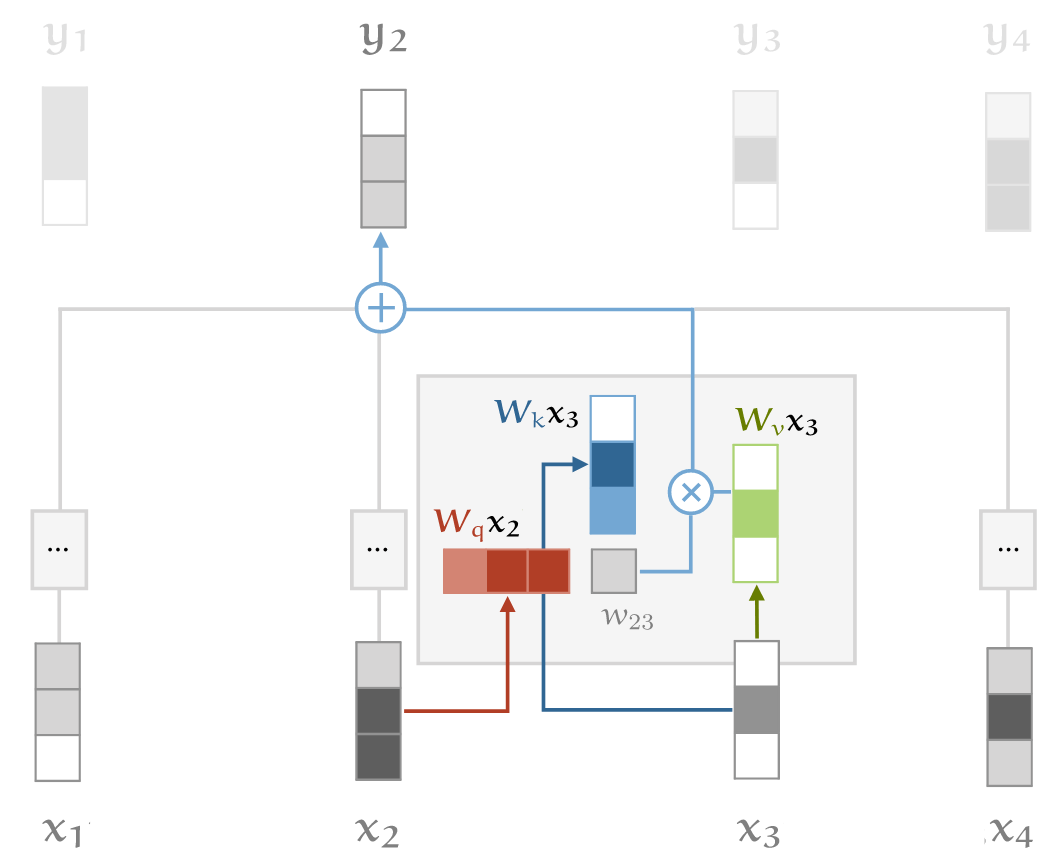self-attention key/query/value transformation 的直观解释

### 3.4.2 对点积做缩放处理（scaling the dot product）

softmax 函数对非常大的输入值敏感。这些 input 会梯度消失，学习变慢甚至完全停止。 由于点积的平均值随着嵌入维度 $k$ 的增加而增大，因此点积送到 softmax 之前进行缩放有助于缓解这个问题。

$w'_{\rc{i}\gc{j}} = {\q_\rc{i}}^T\k_\gc{j}$

$w'_{\rc{i}\gc{j}} = \frac{{\q_\rc{i}}^T\k_\gc{j}} {\sqrt{k}}$

Why $\sqrt{k}$? Imagine a vector in ${\mathbb R^k}$ with values all $c$. Its Euclidean length is $\sqrt{k}c$. Therefore, we are dividing out the amount by which the increase in dimension increases the length of the average vectors

mary,gave,roses,to,susan

• “mary” 表示谁在 “gave”，
• “roses” 表示 “gave” 的是什么，
• “susan” 表示接受者是谁。

#### 需求：输出中嵌入更多信息

This leaves aside how we figure out who gave the roses. We can do that based on prior knowledge about Mary and Susan, encoded in the embeddings. We can also look at the order of the words, but we’ll look at how to achieve that later.

#### 提升 multi-head self-attention 效率：query/key/value 降维

Multi-head self-attention 的缺点是慢，对于 $R$ 头， $R$ 倍。 不过有办法优化：我们可以实现这样的 multi-head self-attention，它既能利用多个 self-attention 提升辨识力， 又与 single-head self-attention 基本一样快。要实现这个目的，每个 head 需要对 query/key/value 降维。 如果输入向量有 $k=256$ 维，我们的模型有 $h=4$ 个 attention head，则降维操作包括：

• 将输入向量乘以一个 256×64 矩阵，这会将 input vector 从 256 维降到 64 维
• 对于每个 head 需要执行 3 次降维：分别针对 query/key/value 的计算。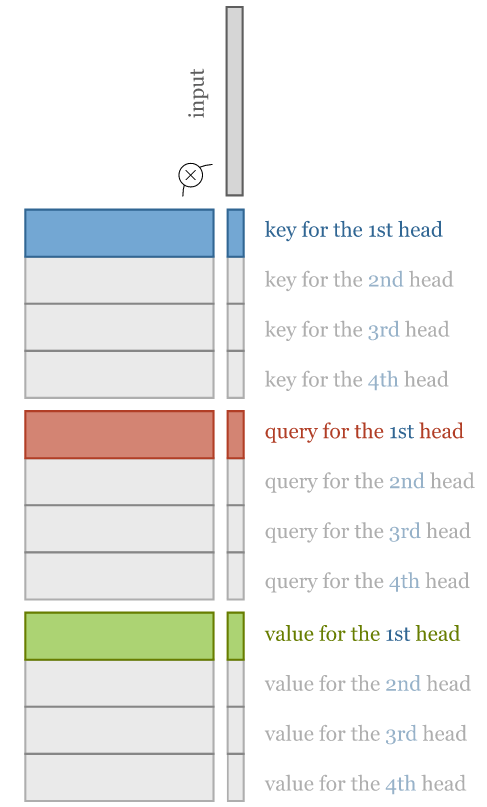To compute multi-head attention efficiently, we combine the computation of the projections down to a lower dimensional representation and the computations of the keys, queries and values into three $k \times k$ matrices.

#### 完整工作流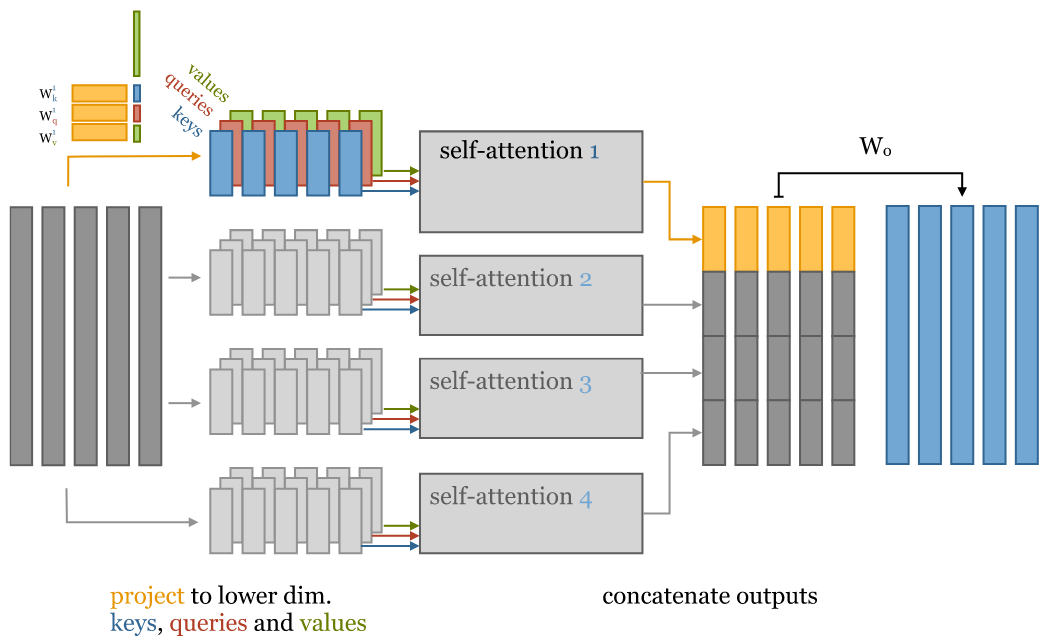1. 原始 256-维 input vector；
2. 输入降维：将 input vector 乘以 256x64 矩阵，降维到 64 维；

注意：对每个 input vector 需要分别针对 query/key/value 降维，总共是 3 遍；

3. 将降维后的 input 分别输入多个并行的 self-attention；
4. 计算得到多个降维之后的 output vector；
5. 对低维度 output vectors 进行拼接，重新回到与 input vectors 一样的维度。

• 权重矩阵 $w_{\rc{i}\gc{j}}$，其中 $i,j \in [0,k]$；
• 3 个平面：query/key/value；

• 每个 head 对应一个 self-attention，每个 self-attention 3 个平面（query/key/value），因此总共 3h 个平面；
• 每个平面的权重矩阵 $w_{\rc{i}\gc{j}}$，其中 $i \in [0,k], j \in [0,k/h]$；## 4.4 self-attention 主要代码实现

import torch
from torch import nn
import torch.nn.functional as F

class SelfAttention(nn.Module):
super().__init__()

assert k % heads == 0 # input vector size 必须是 heads 的整数倍


    # Compute the queries, keys and values for all heads
self.tokeys    = nn.Linear(k, k, bias=False)
self.toqueries = nn.Linear(k, k, bias=False)
self.tovalues  = nn.Linear(k, k, bias=False)

# This will be applied after the multi-head self-attention operation.


def forward(self, x):
b, t, k = x.size()

# 首先，为所有 heads 计算 query/key/value，得到的是完整嵌入维度的 k*k 矩阵
queries = self.toqueries(x)
keys    = self.tokeys(x)
values  = self.tovalues(x)

s = k // h
keys    = keys.view(b, t, h, s)
queries = queries.view(b, t, h, s)
values  = values.view(b, t, h, s)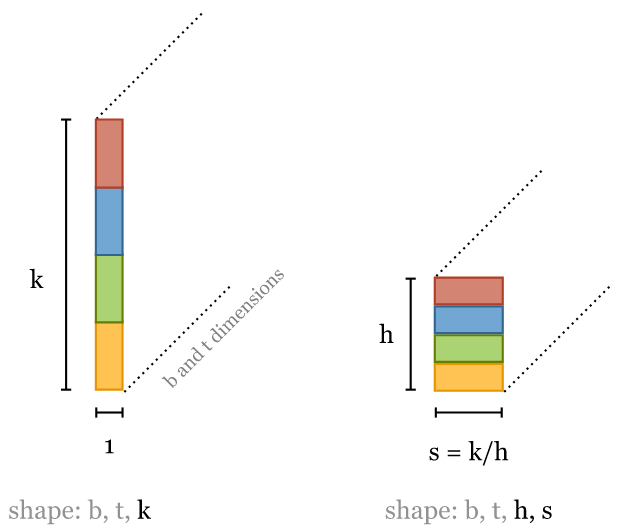# - fold heads into the batch dimension
keys = keys.transpose(1, 2).contiguous().view(b * h, t, s)
queries = queries.transpose(1, 2).contiguous().view(b * h, t, s)
values = values.transpose(1, 2).contiguous().view(b * h, t, s)


You can avoid these calls to contiguous() by using reshape() instead of view() but I prefer to make it explicit when we are copying a tensor, and when we are just viewing it. See this notebook for an explanation of the difference.

    # Get dot product of queries and keys, and scale
dot = torch.bmm(queries, keys.transpose(1, 2)) # -- dot has size (b*h, t, t) containing raw weights
dot = dot / (k ** (1/2))                       # scale the dot product
dot = F.softmax(dot, dim=2)                    # normalize, dot now contains row-wise normalized weights


    out = torch.bmm(dot, values).view(b, h, t, s) # apply the self attention to the values


    # swap h, t back, unify heads
out = out.transpose(1, 2).contiguous().view(b, t, s * h)



The implementation can be made more concise using einsum notation (see an example here).

# 4 基于 multi-head self-attention 实现 transformers

## 4.1 Transformer 定义

transformer 不仅仅是一个 self-attention layer，还是一种架构（architecture）。 如何精确地判断一个东西是或者不是 transformer 还不是很明确，本文采用如下的定义：

## 4.2 Transformer block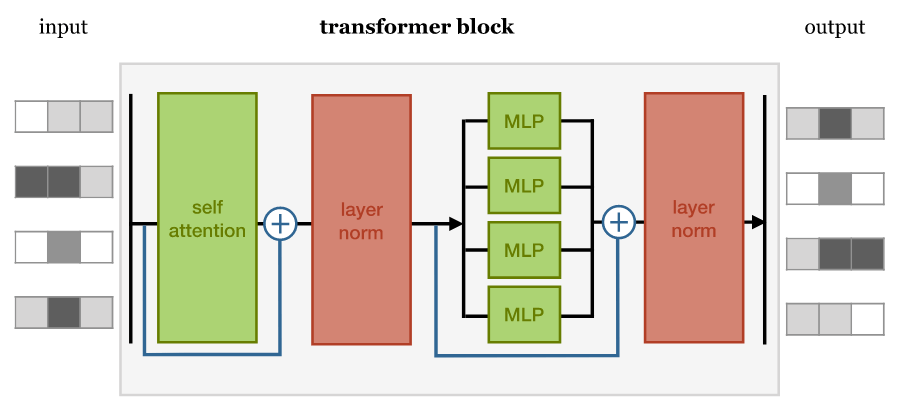1. self-attention 层；
2. 归一化层；
3. 前馈层（feed forward layer），每个 MLP（multi-layer perceptron）分别与每个 input 做运算；
4. 另一个层归一化。

1. 将 self-attention 与局部前馈相结合（combine self-attention with a local feedforward），
2. 添加归一化和残差连接。

class TransformerBlock(nn.Module):
super().__init__()

self.norm1 = nn.LayerNorm(k)
self.norm2 = nn.LayerNorm(k)

self.ff = nn.Sequential(
nn.Linear(k, 4 * k),
nn.ReLU(),
nn.Linear(4 * k, k))

def forward(self, x):
attended = self.attention(x)
x = self.norm1(attended + x)

fedforward = self.ff(x)
return self.norm2(fedforward + x)


## 4.3 文本分类（text classification）transformer

• 数据内容是影评，
• token 化成了单词序列，
• 分类标签是 positivenegative（对电影的正面/负面评价）

• 如何将 input sequence feed 给这个长链，
• 如何对最终 output sequence 进行变换，得到单个分类结果。

### 4.3.1 输出：单个分类结果Overview of a simple sequence classification transformer. The output sequence is averaged to produce a single vector representing the whole sequence. This vector is projected down to a vector with one element per class and softmaxed to produce probabilities.

### 4.3.2 输入：词序敏感（using the positions）

#### 位置编码（position encodings）

$f: {\mathbb N} \to {\mathbb R}^k$

### 4.3.3 基于 Pytorch 实现

class Transformer(nn.Module):
def __init__(self, k, heads, depth, seq_length, num_tokens, num_classes):
super().__init__()

self.num_tokens = num_tokens
self.token_emb = nn.Embedding(num_tokens, k)
self.pos_emb = nn.Embedding(seq_length, k)

# The sequence of transformer blocks that does all the heavy lifting
tblocks = []
for i in range(depth):
self.tblocks = nn.Sequential(*tblocks)

# Maps the final output sequence to class logits
self.toprobs = nn.Linear(k, num_classes)

def forward(self, x):
"""
:param x: A (b, t) tensor of integer values representing words (in some predetermined vocabulary).
:return: A (b, c) tensor of log-probabilities over the classes (where c is the nr. of classes).
"""
# generate token embeddings
tokens = self.token_emb(x)
b, t, k = tokens.size()

# generate position embeddings
positions = torch.arange(t)
positions = self.pos_emb(positions)[None, :, :].expand(b, t, k)

x = tokens + positions # 为什么文本嵌入和位置嵌入相加，没有理论，可能就是实验下来效果不错。
# https://writings.stephenwolfram.com/2023/02/what-is-chatgpt-doing-and-why-does-it-work/
x = self.tblocks(x)

# Average-pool over the t dimension and project to class
# probabilities
x = self.toprobs(x.mean(dim=1))
return F.log_softmax(x, dim=1)


## 4.4 文本生成（text generation）transformer

### 4.4.1 自回归模型和掩码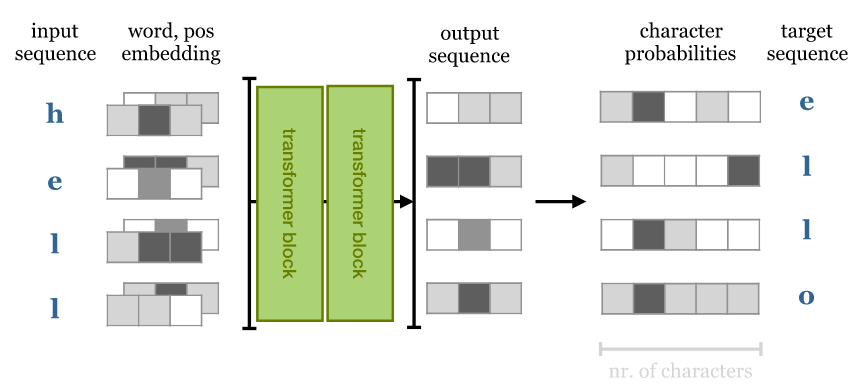• 如果是 RNN 模型，那这就是我们所需做的所有事情， 因为它不能往前看，output $i$ 只依赖 inputs $0$ ~ $i$。
• 而对于 transformer，output 取决于整个 input sequence， 因此预测下一个单词就简单多了，只需从 input 中挑选。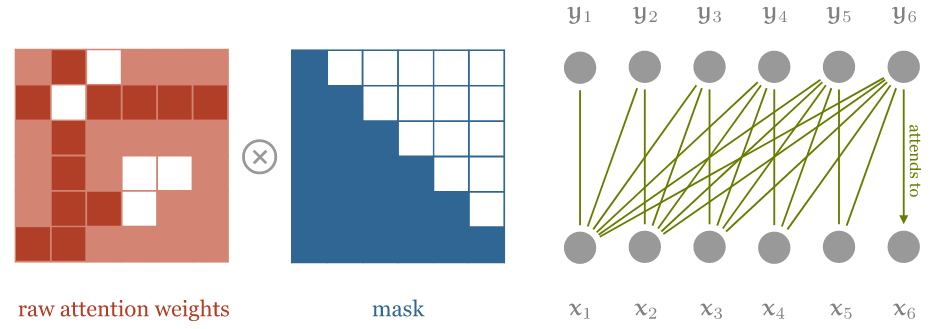dot = torch.bmm(queries, keys.transpose(1, 2))

indices = torch.triu_indices(t, t, offset=1)
dot[:, indices, indices] = float('-inf')

dot = F.softmax(dot, dim=2)


### 4.4.2 训练：基于维基百科数据集 enwik8

1228X Human & Rousseau. Because many of his stories were originally published in long-forgotten magazines and journals, there are a number of [[anthology|anthologies]] by different collators each containing a different selection. His original books have been considered an anthologie in the [[Middle Ages]], and were likely to be one of the most common in the [[Indian Ocean]] in the [[1st century]]. As a result of his death, the Bible was recognised as a counter-attack by the [[Gospel of Matthew]] (1177-1133), and the [[Saxony|Saxons]] of the [[Isle of Matthew]] (1100-1138), the third was a topic of the [[Saxony|Saxon]] throne, and the [[Roman Empire|Roman]] troops of [[Antiochia]] (1145-1148). The [[Roman Empire|Romans]] resigned in [] and [] began to collapse. The [[Saxony|Saxons]] of the [[Battle of Valasander]] reported the y

### 4.4.3 文本生成结果分析

1. 输出的文本中正确使用了维基百科链接标签语法，链接内的文本准确表达了链接主题。
2. 生成的内容也与主题大致一致：生成的文本以圣经和罗马帝国为主题，在不同的地方使用不同的相关术语。
3. 还有一个不那么明显的地方：“Battle of Valasander”，这场“战争”似乎是这个神经网络自己杜撰的

## 4.5 设计考虑：Transformer 与 RNN/卷积 对比

transformer 之前，最先进的架构是 RNN（通常是 LSTM 或 GRU），但它们存在一些问题。

RNN 展开（unrolled）后长这样：RNN 最大的问题是级联（recurrent connection）： 虽然它使得信息能沿着 input sequence 一路传导， 但也意味着在计算出 $i-1$ 单元之前，无法计算出 $i$ 单元的输出。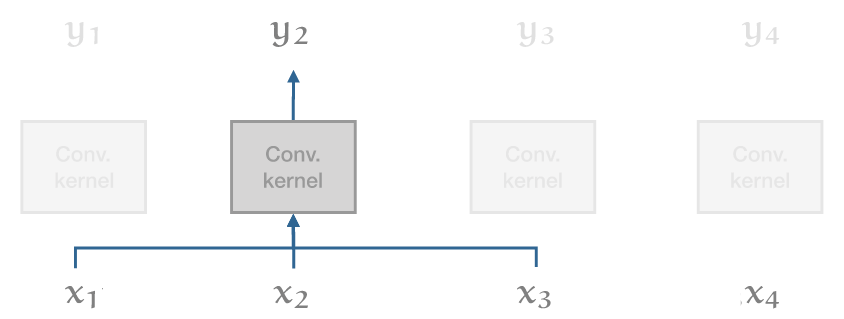Transformer 试图兼顾二者的优点

• 可以像对彼此相邻的单词一样，轻松地对输入序列的整个范围内的依赖关系进行建模（事实上，如果没有位置向量，二者就没有区别）；
• 同时，避免 recurrent connections，因此整个模型可以用非常高效的 feed forward 方式计算。

Transformer 的其余设计主要基于一个考虑因素 —— 深度 —— 大多数选择都是训练大量 transformer block 层，例如，transformer 中只有两个非线性的地方

1. self-attention 中的 softmax；
2. 前馈层中的 ReLU。

I suppose the layer normalization is also nonlinear, but that is one nonlinearity that actually helps to keep the gradient stable as it propagates back down the network.

# 5 历史包袱

## 5.1 为什么叫 self-attention？

• 我们把 input 称为 values（因为它们是实实在在的值，我们将基于这些值计算输出）；
• 然后，一些（trainable）机制为每个 value 分配一个 key
• 最后，对每个 output，一些其他机制分配一个 query。

Attention（注意力）模型是 key-value store 模型的宽松版：

• store 中的每个 key 都能在某种程度上（而不是精确 100% 或 0%）匹配到 query；
• 另外，query 返回的也不是单个 value，而是所有 value，我们根据每个 key 与 query 匹配的程度对相应 value 取一个加权和

self-attention 的重大突破在于，attention 本身就是一种足够强大的机制，能完成所有学习。 正如作者所说，Attention is all you need

• Key/value/query 都来自同一个 input vector（只是各自经过了略微不同的线性变换）；
• 他们关注自己（attend to themselves），因此叫 self-attention；
• 这种 self-attention 经过多层堆叠之后，就能提供足够的非线性和表征能力（nonlinearity and representational power）来学习非常复杂的功能。

## 5.2 最初的 transformer: encoders and decoders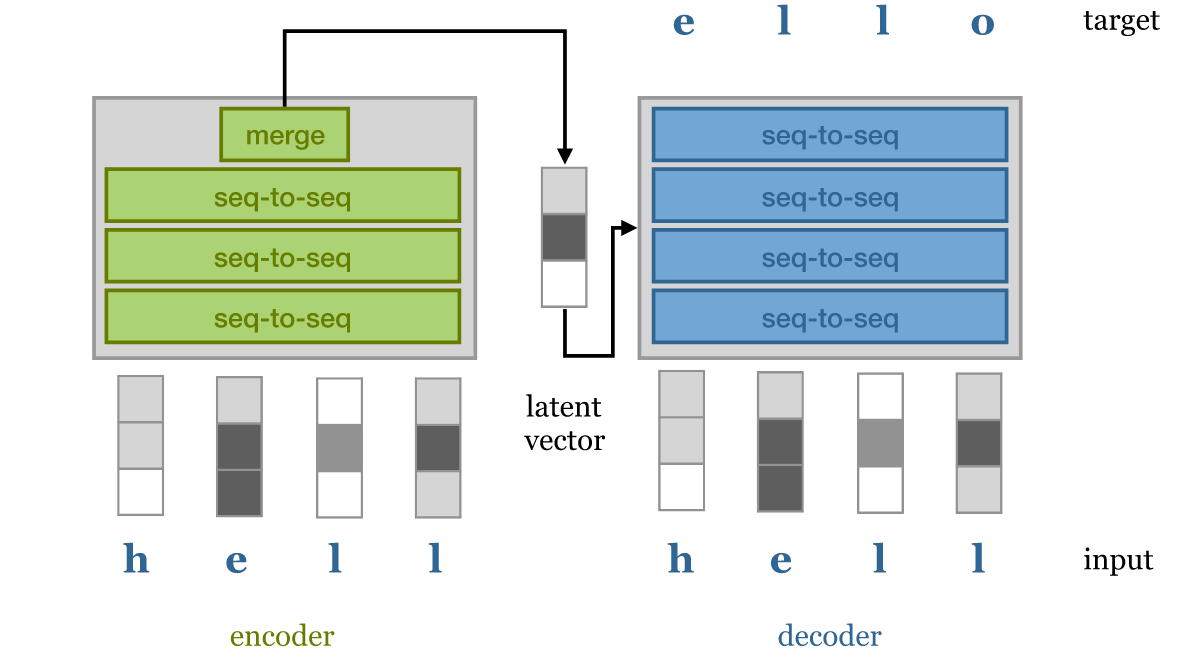encoder-decoder 模型

Encoder 获取输入序列并将整个 sequence 映射为一个 latent representations， 这可以是一系列 latent vectors，也可以是如上图中的单个向量。 然后将该向量传递给 decoder，后者将其解码为期望的目标序列（例如，同一句话的另一种语言表示）。

Teacher forcing 指的是允许 decoder 访问 input 的技术 —— 但以自回归（autoregressive）的方式。 也就是说， decoder 基于 latent vectors 和它自己已经生成的单词，逐单词生成输出句子。 这减轻了 latent representations 的一些压力：

• decoder 可以使用逐词采样（word-by-word sampling）来处理语法（syntax and grammar）等低级结构，
• 而使用 latent vectors 来 capture 更高级别的语义结构（semantic structure）。

# 6 现代 transformers

## 6.1 Google BERT：340M 参数

BERT (Bidirectional Encoder Representations from Transformers) 是首批证明 transformer 可以在各种基于语言的任务上 （question answering, sentiment classification or classifying whether two sentences naturally follow one another） 达到人类水平的模型之一。

BERT 由一些与本文描述的类似的简单 transformer block 堆叠而成，然后在一个大型通用领域语料库上进行预训练， 该语料库由包含 8 亿个（800M）单词的英文书籍（现代作品，from unpublished authors） 和包含 25 亿（2.5B）个单词英文维基百科文章（去掉了 markup）组成。

A certain number of words in the input sequence are: masked out, replaced with a random word or kept as is. The model is then asked to predict, for these words, what the original words were. Note that the model doesn't need to predict the entire denoised sentence, just the modified words. Since the model doesn't know which words it will be asked about, it learns a representation for every word in the sequence.
Next sequence classification
Two sequences of about 256 words are sampled that either (a) follow each other directly in the corpus, or (b) are both taken from random places. The model must then predict whether a or b is the case.

BERT uses WordPiece tokenization, which is somewhere in between word-level and character level sequences. It breaks words like walking up into the tokens walk and ##ing. This allows the model to make some inferences based on word structure: two verbs ending in -ing have similar grammatical functions, and two verbs starting with walk- have similar semantic function.

The input is prepended with a special </span> token. The output vector corresponding to this token is used as a sentence representation in sequence classification tasks like the next sentence classification (as opposed to the global average pooling over all vectors that we used in our classification model above).

After pretraining, a single task-specific layer is placed after the body of transformer blocks, which maps the general purpose representation to a task specific output. For classification tasks, this simply maps the first output token to softmax probabilities over the classes. For more complex tasks, a final sequence-to-sequence layer is designed specifically for the task.

The whole model is then re-trained to finetune the model for the specific task at hand.

In an ablation experiment, 作者展示了与之前的模型相比，最大的改进来自 BERT 的双向特性（bidirectional nature）。 之前的模型，例如 GPT，使用的是 autoregressive mask，只允许 attention 使用前面的 token。 在 BERT 中，all attention is over the whole sequence，这是性能提升的主要来源。

## 6.2 OpenAI GPT-2：1.5B 参数

They show state-of-the art performance on many tasks. On the wikipedia compression task that we tried above, they achieve 0.93 bits per byte.

GPT-2 是第一个真正进入主流新闻的 transformer 模型，原因是 GPT-2 可以生成看起来足够可信的文本，如果 2016 年有这种技术， 那当年美国总统大选中出现的那种大规模假新闻活动只需要一个人就能完成了。

GPT-2 第一个技巧是构建一个新的高质量数据集

• 虽然 BERT 使用了高质量的数据，但数据的来源（精心编写的书籍和维基百科文章）在写作风格上缺乏多样性；
• 为了在不牺牲质量的前提下收集更多不同的数据，作者使用社交媒体网站 Reddit 上的链接来收集大量文本。

GPT2 本质上是一个语言生成模型（language generation model）， 因此像我们自己设计的 text generation transformer 一样，它也使用了 masked self-attention。 它使用字节对编码（byte-pair encoding）来 tokenize the language， 这与 WordPiece encoding 一样将单词拆分为比“比单词短、比单个字母长”的 tokens

GPT2 与我们的 text generation transformer 非常相似，只有很小的层级顺序差异，以及增加了训练深度。 最大的模型使用 48 个 transformer block，序列长度为 1024，嵌入维度为 1600，总共 15 亿个（1.5B）参数

GPT2 在很多任务上都表现出了最先进的性能。在上面提到的维基百科压缩任务中，它取得了每字节 0.93 位的压缩效率。

## 6.3 Transformer-XL

While the transformer represents a massive leap forward in modeling long-range dependency, the models we have seen so far are still fundamentally limited by the size of the input. Since the size of the dot-product matrix grows quadratically in the sequence length, this quickly becomes the bottleneck as we try to extend the length of the input sequence. Transformer-XL is one of the first succesful transformer models to tackle this problem.

During training, a long sequence of text (longer than the model could deal with) is broken up into shorter segments. Each segment is processed in sequence, with self-attention computed over the tokens in the curent segment and the previous segment. Gradients are only computed over the current segment, but information still propagates as the segment window moves through the text. In theory at layer $n$, information may be used from $n$ segments ago.

A similar trick in RNN training is called truncated backpropagation through time. We feed the model a very long sequence, but backpropagate only over part of it. The first part of the sequence, for which no gradients are computed, still influences the values of the hidden states in the part for which they are.

To make this work, the authors had to let go of the standard position encoding/embedding scheme. Since the position encoding is absolute, it would change for each segment and not lead to a consistent embedding over the whole sequence. Instead they use a relative encoding. For each output vector, a different sequence of position vectors is used that denotes not the absolute position, but the distance to the current output.

This requires moving the position encoding into the attention mechanism (which is detailed in the paper). One benefit is that the resulting transformer will likely generalize much better to sequences of unseen length.

## 6.4 Sparse transformers

Sparse transformers tackle the problem of quadratic memory use head-on. Instead of computing a dense matrix of attention weights (which grows quadratically), they compute the self-attention only for particular pairs of input tokens, resulting in a sparse attention matrix, with only $n\sqrt{n}$ explicit elements.

This allows models with very large context sizes, for instance for generative modeling over images, with large dependencies between pixels. The tradeoff is that the sparsity structure is not learned, so by the choice of sparse matrix, we are disabling some interactions between input tokens that might otherwise have been useful. However, two units that are not directly related may still interact in higher layers of the transformer (similar to the way a convolutional net builds up a larger receptive field with more convolutional layers).

Beyond the simple benefit of training transformers with very large sequence lengths, the sparse transformer also allows a very elegant way of designing an inductive bias. We take our input as a collection of units (words, characters, pixels in an image, nodes in a graph) and we specify, through the sparsity of the attention matrix, which units we believe to be related. The rest is just a matter of building the transformer up as deep as it will go and seeing if it trains.

# 7 大型模型优化

• 对于序列长度 $t$，这是一个包含 $t^2$ 个元素的稠密矩阵。
• 在标准的 32 位精度下，当 $t=1000$ 时，16 矩阵作为一个 batch，这个 batch 占用大约 250Mb 的显存。
• 由于我们每个 self-attention 操作至少需要四个层（在 softmax 之前和之后，加上它们的梯度），这限制了在标准 12Gb GPU 中最多只能使用 12 层。

# 8 结束语

Transformer 很可能是未来几十年占主导地位的最简单机器学习架构。作为从业者，有充分的理由关注它们。

• 基本 transformer 是一个 set-to-set 模型。 只要数据是基本单位组成的集合（a set of units），就可以应用 transformer；
• 数据的其他信息（如局部结构），可以通过位置嵌入或通过 manipulate 注意力矩阵的结构（使其稀疏或屏蔽部分）来添加， 这在多模态学习（multi-modal learning）中特别有用。例如，可以轻松地将带字幕的图像 分解为像素集合和字符集合，然后设计一些精巧的嵌入和稀疏结构来帮助模型组合和对齐二者。 如果我们将关于某一领域的全部知识组合成一个关系型结构（relational structure）， 如多模态知识图谱（multi-modal knowledge graph，），那就可以使用简单的 transformer block 在多模态单元之间传播信息， 然后通过稀疏结构控制与哪些单元直接交互。

# 参考资料

1. The illustrated transformer, Jay Allamar.
2. The annotated transformer, Alexander Rush.
3. The knowledge graph as the default data model for learning on heterogeneous knowledge Xander Wilcke, Peter Bloem, Victor de Boer
4. Matrix factorization techniques for recommender systems Yehuda Koren et al.